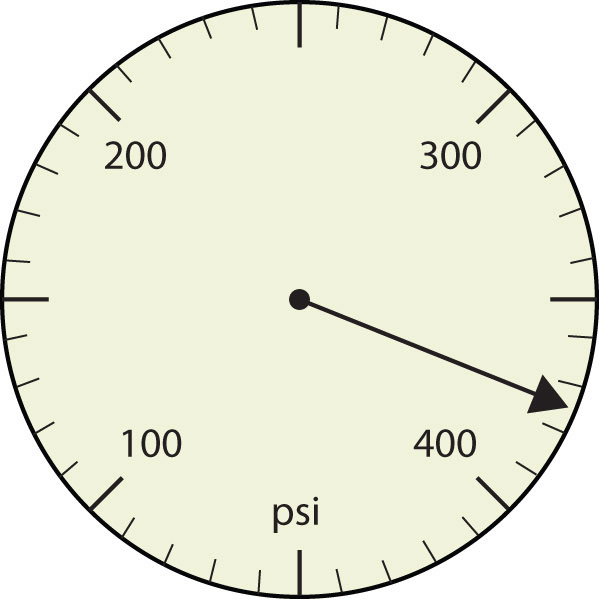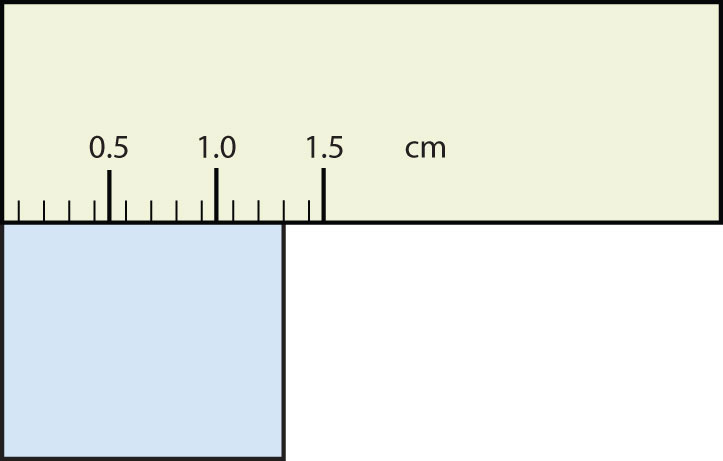QUESTION ANSWER 1. Express each measurement to the correct number of significant figures.1. 375 psi 1.30 cm 3. How many significant figures do these numbers have? 23 23.0 0.00023 0.0002302 3. two three two four 5. How many significant figures do these numbers have? 765,890 765,890.0 1.2000 × 105 0.0005060 5. five seven five four 7. Compute and express each answer with the proper number of significant figures, rounding as necessary. 56.0 + 3.44 = ? 0.00665 + 1.004 = ? 45.99 − 32.8 = ? 45.99 − 32.8 + 75.02 = ? 7. 59.4 1.011 13.2 88.2 9. Compute and express each answer with the proper number of significant figures, rounding as necessary. 56.7 × 66.99 = ? 1.000 ÷ 77 = ? 1.000 ÷ 77.0 = ? 6.022 × 1.89 = ? 9. 3.80 × 103 0.013 0.0130 11.4 11. Write the number 87,449 in scientific notation with four significant figures. Write the number 0.000066600 in scientific notation with five significant figures. 11. 8.745 × 104 6.6600 × 10−5 13. Perform each calculation and limit each answer to three significant figures. 67,883 × 0.004321 = ? (9.67 × 103) × 0.0055087 = ? 13. 293 53.3